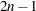Special SAS Data Sets
 TYPE=TREE Data Sets

Some clustering procedures produce TYPE=TREE data sets. For example, in PROC CLUSTER, a TYPE=TREE data set contains one observation for each observation in the input data set, plus one observation for each cluster of two or more observations (that is, one observation for each node of the cluster tree). The total number of output observations is usually, whereis the number of input observations. The density methods might produce fewer output observations when the number of clusters cannot be reduced to one.

In PROC VARCLUS, the OUTTREE= data set contains one observation for each variable clustered plus one observation for each cluster of two or more variables—that is, one observation for each node of the cluster tree. The total number of output observations is betweenand, whereis the number of variables clustered. See Chapter 29, The CLUSTER Procedure, and Chapter 94, The VARCLUS Procedure, for details.Previous Page | Next Page | Top of Page